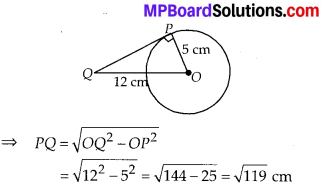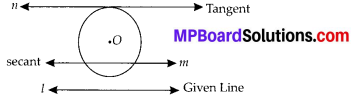# MP Board Class 10th Maths Solutions Chapter 10 Circles Ex 10.1

In this article, we will share MP Board Class 10th Maths Book Solutions Chapter 10 Circles Ex 10.1 Pdf, These solutions are solved subject experts from the latest edition books.

## MP Board Class 10th Maths Solutions Chapter 10 Circles Ex 10.1

Question 1.
How many tangents can a circle have?
Solution:
A circle can have an infinite number of tangents.

Question 2.
Fill in the blanks :
(i) A tangent to a circle intersects it in _________ point(s).
(ii) A line intersecting a circle in two points is called a ______.
(iii) A circle can have ______ parallel tangents at the most.
(iv) The common point of a tangent to a circle and the circle is called _______.
Solution:
(i) Exactly one
(ii) Secant
(iii) Two
(iv) Point of contactQuestion 3.
A tangent PQ at a point P of a circle of radius 5 cm meets a line through the centre O at a point Q so that OQ = 12 cm. Length PQ is
(A) 12 cm
(B) 13 cm
(C) 8.5 cm
(D) $$\sqrt{119}$$ cm
Solution:
In right ∆QPO,
OQ2 = OP2 + PQ2Question 4.
Draw a circle and two lines parallel to a given line such that one is a tangent and the other, a secant to the circle.
Solution:
We have the required figure, as shownHere, l is the given line and a circle with centre O is drawn.
Line n is drawn which is parallel to l and tangent to the circle. Also, m is drawn parallel to line 1 and is a secant to the circle.